# NCERT solutions for Mathematics Exemplar Class 10 chapter 9 - Circles [Latest edition]

#### Chapters## Chapter 9: Circles

Exercise 9.1Exercise 9.2Exercise 9.3Exercise 9.4
Exercise 9.1 [Pages 102 - 104]

### NCERT solutions for Mathematics Exemplar Class 10 Chapter 9 Circles Exercise 9.1 [Pages 102 - 104]

#### Choose the correct alternative:

Exercise 9.1 | Q 1 | Page 102

If radii of two concentric circles are 4 cm and 5 cm, then the length of each chord of one circle which is tangent to the other circle is ______.

• 3 cm

• 6 cm

• 9 cm

• 1 cm

Exercise 9.1 | Q 2 | Page 102

In figure, if ∠AOB = 125°, then ∠COD is equal to ______.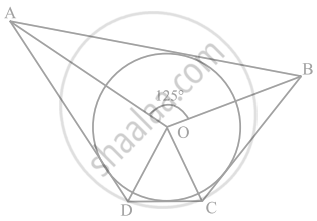• 62.5°

• 45°

• 35°

• 55°

Exercise 9.1 | Q 3 | Page 102

If figure AB is a chord of the circle and AOC is its diameter such that ∠ACB = 50°. If AT is the tangent to the circle at point A, the ∠BAT is equal to ______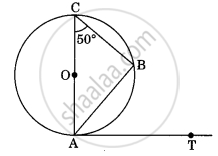• 65°

• 60°

• 50°

• 40°

Exercise 9.1 | Q 4 | Page 103

From a point P which is at a distance of 13 cm from the point O of a circle of radius 5 cm, the pair of tangents PQ and PR to the circle are drawn. Then the area of the quadrilateral PQOR is ______

• 60 cm2

• 65 cm2

• 30 cm2

• 32.5 cm2

Exercise 9.1 | Q 5 | Page 103

At one end A of a diameter AB of a circle of radius 5 cm, tangent XAY is drawn to the circle. The length of the chord CD parallel to XY and at a distance of 8 cm from A is ______

• 4 cm

• 5 cm

• 6 cm

• 8 cm

Exercise 9.1 | Q 6 | Page 103

In figure, AT is a tangent to the circle with centre O such that OT = 4 cm and ∠OTA = 30°. Then AT is equal to ______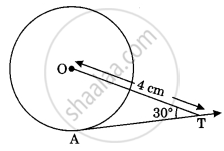• 4 cm

• 2 cm

• 2sqrt3 cm

• 4sqrt3 cm

Exercise 9.1 | Q 7 | Page 103

In the following figure, if O is the centre of a circle, PQ is a chord and the tangent PR at P makes an angle of 50° with PQ, then ∠POQ is equal to ______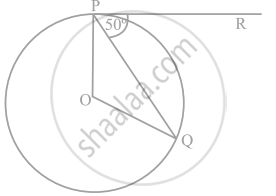• 100°

• 80°

• 90°

• 75°

Exercise 9.1 | Q 8 | Page 103

In the figure, if PA and PB are tangents to the circle with centre O such that ∠APB = 50°, then ∠AOB is equal to ______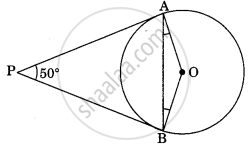• 25°

• 30°

• 40°

• 50°

Exercise 9.1 | Q 9 | Page 104

If two tangents inclined at an angle of 60° are drawn to a circle of radius 3 cm the length of each tangent is equal to ______

• (3sqrt(3))/2  cm

• 6 cm

• 3 cm

• 3sqrt(3) cm

Exercise 9.1 | Q 10 | Page 104

In figure, if PQR is the tangent to a circle at Q whose centre is O, AB is a chord parallel to PR and ∠BQR = 70°, then ∠AQB is equal to ______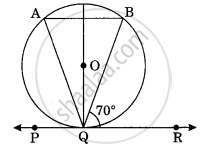• 20°

• 40°

• 35°

• 45°

Exercise 9.2 [Pages 104 - 106]

### NCERT solutions for Mathematics Exemplar Class 10 Chapter 9 Circles Exercise 9.2 [Pages 104 - 106]

#### State whether the following statement is True or False:

Exercise 9.2 | Q 1 | Page 104

If a chord AB subtends an angle of 60° at the centre of a circle, then angle between the tangents at A and B is also 60°.

• True

• False

Exercise 9.2 | Q 2 | Page 104

The length of tangent from an external point on a circle is always greater than the radius of the circle.

• True

• False

Exercise 9.2 | Q 3 | Page 105

The length of tangent from an external point P on a circle with centre O is always less than OP.

• True

• False

Exercise 9.2 | Q 4 | Page 105

The angle between two tangents to a circle may be 0°.

• True

• False

Exercise 9.2 | Q 5 | Page 105

If angle between two tangents drawn from a point P to a circle of radius a and centre O is 90°, then OP = asqrt(2)

• True

• False

Exercise 9.2 | Q 6 | Page 105

If angle between two tangents drawn from a point P to a circle of radius a and centre O is 60°, then OP = asqrt(3)

• True

• False

Exercise 9.2 | Q 7 | Page 105

The tangent to the circumcircle of an isosceles triangle ABC at A, in which AB = AC, is parallel to BC.

• True

• False

Exercise 9.2 | Q 8 | Page 106

If a number of circles touch a given line segment PQ at a point A, then their centres lie on the perpendicular bisector of PQ.

• True

• False

Exercise 9.2 | Q 9 | Page 106

If a number of circles pass through the endpoints P and Q of a line segment PQ, then their centres lie on the perpendicular bisector of PQ.

• True

• False

Exercise 9.2 | Q 10 | Page 106

AB is a diameter of a circle and AC is its chord such that ∠BAC = 30°. If the tangent at C intersects AB extended at D, then BC = BD.

• True

• False

Exercise 9.3 [Pages 107 - 108]

### NCERT solutions for Mathematics Exemplar Class 10 Chapter 9 Circles Exercise 9.3 [Pages 107 - 108]

Exercise 9.3 | Q 1 | Page 107

Out of the two concentric circles, the radius of the outer circle is 5 cm and the chord AC of length 8 cm is a tangent to the inner circle. Find the radius of the inner circle.

Exercise 9.3 | Q 2 | Page 107

Two tangents PQ and PR are drawn from an external point to a circle with centre O. Prove that QORP is a cyclic quadrilateral.

Exercise 9.3 | Q 3 | Page 107

If from an external point B of a circle with centre O, two tangents BC and BD are drawn such that ∠DBC = 120°, prove that BC + BD = BO, i.e., BO = 2BC.

Exercise 9.3 | Q 4 | Page 107

Prove that the centre of a circle touching two intersecting lines lies on the angle bisector of the lines .

Exercise 9.3 | Q 5 | Page 107

In the figure, AB and CD are common tangents to two circles of unequal radi. Prove that AB = CD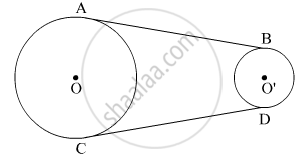Exercise 9.3 | Q 6 | Page 107

In Question 5 above, if radii of the two circles are equal, prove that AB = CD.

Exercise 9.3 | Q 7 | Page 107

In figure, common tangents AB and CD to two circles intersect at E. Prove that AB = CD.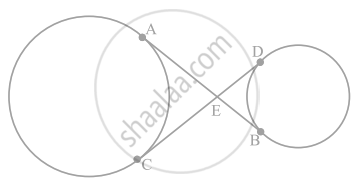Exercise 9.3 | Q 8 | Page 107

A chord PQ of a circle is parallel to the tangent drawn at a point R of the circle. Prove that R bisects the arc PRQ.

Exercise 9.3 | Q 9 | Page 108

Prove that the tangents drawn at the ends of a chord of a circle make equal angles with the chord.

Exercise 9.3 | Q 10 | Page 108

Prove that a diameter AB of a circle bisects all those chords which are parallel to the tangent at the point A.

Exercise 9.4 [Pages 110 - 112]

### NCERT solutions for Mathematics Exemplar Class 10 Chapter 9 Circles Exercise 9.4 [Pages 110 - 112]

Exercise 9.4 | Q 1 | Page 110

If a hexagon ABCDEF circumscribe a circle, prove that AB + CD + EF = BC + DE + FA.

Exercise 9.4 | Q 2 | Page 110

Let s denote the semi-perimeter of a triangle ABC in which BC = a, CA = b, AB = c. If a circle touches the sides BC, CA, AB at D, E, F, respectively, prove that BD = s – b.

Exercise 9.4 | Q 3 | Page 110

From an external point P, two tangents, PA and PB are drawn to a circle with centre O. At one point E on the circle tangent is drawn which intersects PA and PB at C and D, respectively. If PA = 10 cm, find the the perimeter of the triangle PCD.

Exercise 9.4 | Q 4 | Page 110

If AB is a chord of a circle with centre O, AOC is a diameter and AT is the tangent at A as shown in figure. Prove that ∠BAT = ∠ACBExercise 9.4 | Q 5 | Page 110

Two circles with centres O and O' of radii 3 cm and 4 cm, respectively intersect at two points P and Q such that OP and O'P are tangents to the two circles. Find the length of the common chord PQ.

Exercise 9.4 | Q 6 | Page 110

In a right triangle ABC in which ∠B = 90°, a circle is drawn with AB as diameter intersecting the hypotenuse AC and P. Prove that the tangent to the circle at P bisects BC.

Exercise 9.4 | Q 7 | Page 110

In figure, tangents PQ and PR are drawn to a circle such that ∠RPQ = 30°. A chord RS is drawn parallel to the tangent PQ. Find the ∠RQS.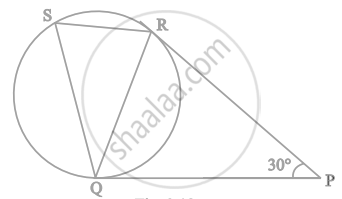Exercise 9.4 | Q 8 | Page 111

AB is a diameter and AC is a chord of a circle with centre O such that ∠BAC = 30°. The tangent at C intersects extended AB at a point D. Prove that BC = BD.

Exercise 9.4 | Q 9 | Page 111

Prove that the tangent drawn at the mid-point of an arc of a circle is parallel to the chord joining the end points of the arc.

Exercise 9.4 | Q 10 | Page 111

In figure the common tangent, AB and CD to two circles with centres O and O' intersect at E. Prove that the points O, E, O' are collinear.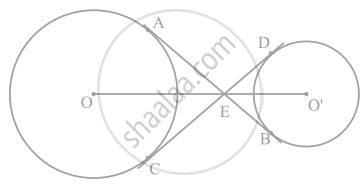Exercise 9.4 | Q 11 | Page 111

In figure. O is the centre of a circle of radius 5 cm, T is a point such that OT = 13 cm and OT intersects the circle at E. If AB is the tangent to the circle at E, find the length of AB.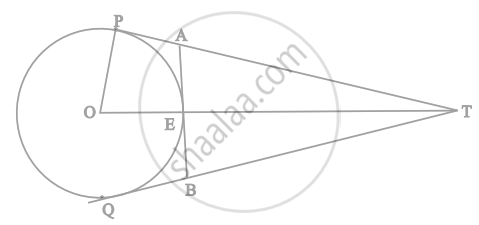Exercise 9.4 | Q 12 | Page 112

The tangent at a point C of a circle and a diameter AB when extended intersect at P. If ∠PCA = 110°, find ∠CBA see figure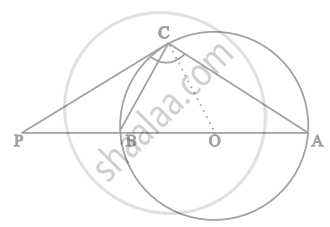Exercise 9.4 | Q 13 | Page 112

If an isosceles triangle ABC, in which AB = AC = 6 cm, is inscribed in a circle of radius 9 cm, find the area of the triangle

Exercise 9.4 | Q 14 | Page 112

A is a point at a distance 13 cm from the centre O of a circle of radius 5 cm. AP and AQ are the tangents to the circle at P and Q. If a tangent BC is drawn at a point R lying on the minor arc PQ to intersect AP at B and AQ at C, find the perimeter of the ∆ABC.

## Chapter 9: Circles

Exercise 9.1Exercise 9.2Exercise 9.3Exercise 9.4## NCERT solutions for Mathematics Exemplar Class 10 chapter 9 - Circles

NCERT solutions for Mathematics Exemplar Class 10 chapter 9 (Circles) include all questions with solution and detail explanation. This will clear students doubts about any question and improve application skills while preparing for board exams. The detailed, step-by-step solutions will help you understand the concepts better and clear your confusions, if any. Shaalaa.com has the CBSE Mathematics Exemplar Class 10 solutions in a manner that help students grasp basic concepts better and faster.

Further, we at Shaalaa.com provide such solutions so that students can prepare for written exams. NCERT textbook solutions can be a core help for self-study and acts as a perfect self-help guidance for students.

Concepts covered in Mathematics Exemplar Class 10 chapter 9 Circles are Tangent to a Circle, Number of Tangents from a Point on a Circle, Concept of Circle - Centre, Radius, Diameter, Arc, Sector, Chord, Segment, Semicircle, Circumference, Interior and Exterior, Concentric Circles, Concept of Circle - Centre, Radius, Diameter, Arc, Sector, Chord, Segment, Semicircle, Circumference, Interior and Exterior, Concentric Circles.

Using NCERT Class 10 solutions Circles exercise by students are an easy way to prepare for the exams, as they involve solutions arranged chapter-wise also page wise. The questions involved in NCERT Solutions are important questions that can be asked in the final exam. Maximum students of CBSE Class 10 prefer NCERT Textbook Solutions to score more in exam.

Get the free view of chapter 9 Circles Class 10 extra questions for Mathematics Exemplar Class 10 and can use Shaalaa.com to keep it handy for your exam preparation